×

Search anything:

# Implementing Queue using Linked list

#### Data Structures queue linked listGet this book -> Problems on Array: For Interviews and Competitive Programming

Reading time: 35 minutes | Coding time: 12 minutes

A queue is an ordered collection of items where the addition of new items happens at one end, called the “rear,” and the removal of existing items occurs at the other end, commonly called the “front.” The first element to be inserted is the first one to be deleted. Hence, it is called First in First out (FIFO) or Last in Last out (LILO) list.

Real life example of queues are people standing in queue to buying tickets, ants walking in queue.### Why we want to implement Queue using linked list?

The major problem with the queue implemented using array is, It will work for only fixed number of data values. That means, the amount of data must be specified in the beginning itself.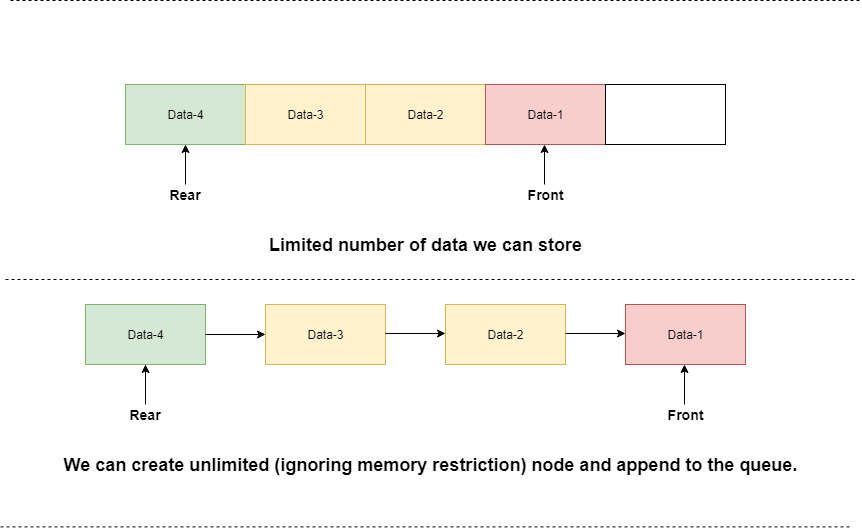Queue using array is not suitable when we do not know the size of data which we are going to use.

A queue data structure can be implemented using linked list data structure. The queue which is implemented using linked list can work for unlimited number of values.

That means, queue using linked list can work for variable size of data (No need to fix the size at beginning of the implementation). The Queue implemented using linked list can organize as many data values as we want.

We will implement Queue using linked list. Queues maintain two data pointers:

• FRONT: to know first inserted element
• REAR to know last inserted element.

Queue is very simple data structure, you only have to manipulate FRONT and REAR to get Queue property.

# Basic Operation:

Following are basic operations of Queue:

Main Queue Operations:
1)EnQueue(): Inserts an element at the rear of the Queue.
2)DeQueue(): Remove and return the front element of the Queue.

Auxiliary Queue operation:

1)Front(): Display the data front of the Queue.
2)QueueSize(): Returns the number of elements stored in the Queue.
3)display(): Display elements of queue.

There are many different operations that we can implement.
For ex.:- Merging of Two Queue,Sorting of Queue etc..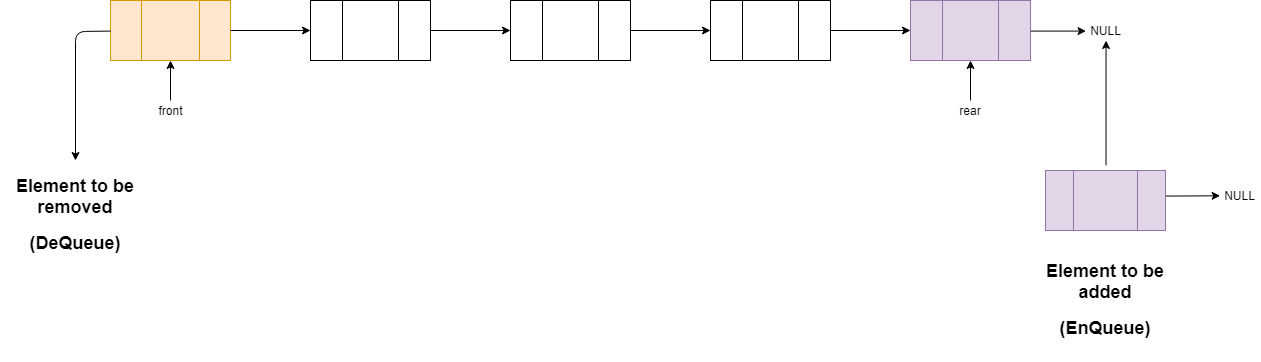• Let's see implementation of Queue and its operations,with example.
For linked list we will make structure of Node using struct keyword.In which we here taking int data and one pointer of that struct to point another Node.
• First we will take two pointers of that struct Node, front=NULL and rear=NULL.

### EnQueue

Inserting an element in Queue.

• Initially both of our pointers pointing to the front and rear are NULL.
• Now,If We enter the 12 in Queue we will checking weather there is any element present or not.If rear is NULL,We make our front and rear pointers to point our newly inserted Node with having data '12'.
• Now,again if we want to enter new data,Let say 45.We will check for rear NULL or not.Now our rear would be pointing to '12'.So 45 will added next to the rear Node and 45 would be our rear Node.
• Same way if i add 26, it would be added after 45 and rear will point to the Node having data '26'.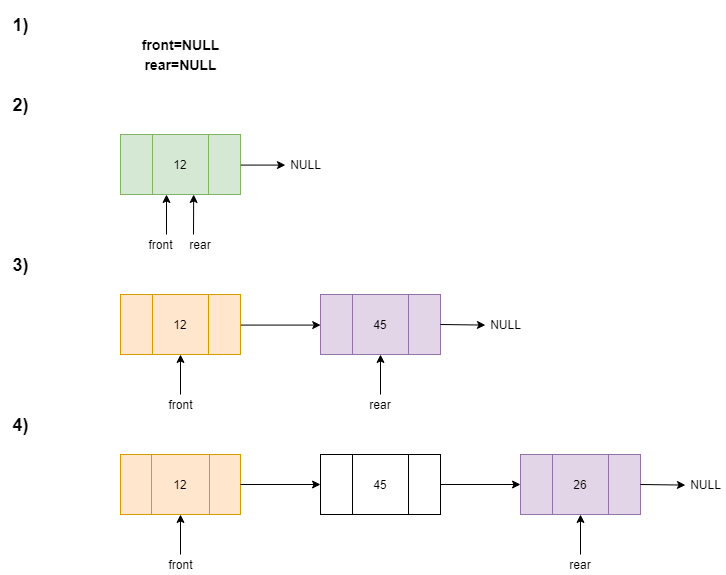Pseudocode:

``````Step 1 : Create a newNode with given value and set 'newNode → next' to NULL.
Step 2 : Check whether queue is Empty (rear == NULL)
Step 3 : If it is Empty then, set front = newNode and rear = newNode.
Step 4 : If it is Not Empty then, set rear → next = newNode and rear = newNode.
``````

### DeQueue

Deleting an element from Queue.

• Now, by doing three EnQueue operation we will be having Queue having 3 elements as shown in figure.
• First we check that front is NULL?If it is then Queue is empty we can not perform DeQueue operation.But here front will be pointing to '12'.
• So if we want to delete element from Queue. As our Queue is FIFO(first in first out) 12 is first inserted, so 12 will be removed.Our front pointer will be pointing to the '12'.To,remove it first we make our front pointer to point the element inserted after '12'.And then simply we free the memory.
• As simple as that 45 and 26 will be removed if we perform two more DeQueue operation.And again our Queue will be empty(rear and front will be eqal to NULL).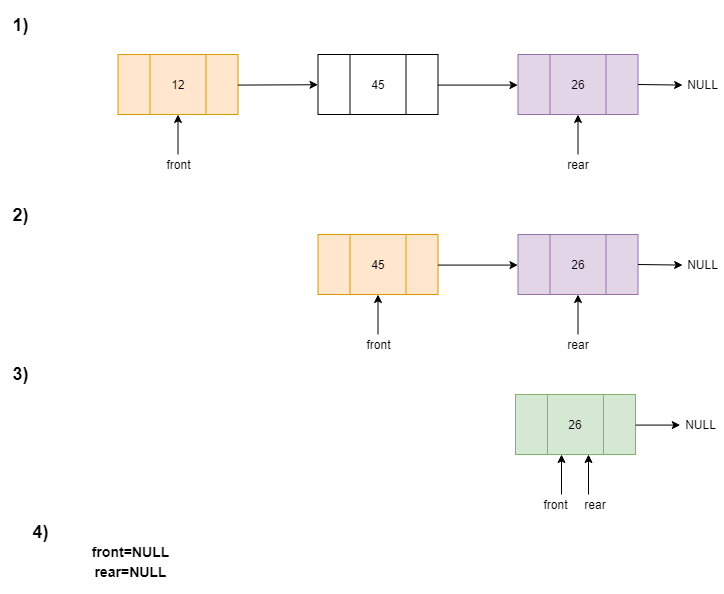``````Step 1 : Check whether queue is Empty (front == NULL).
Step 2 : If it is Empty, then display "Queue is Empty!!! Deletion is not possible!!!" and terminate from the function
Step 3 : If it is Not Empty then, define a Node pointer 'temp' and set it to 'front'.
Step 4 : Then set 'front = front → next' and delete 'temp' (free(temp)).

``````

### Display

• To display all elements present in Queue, we will take one temp(you can give whatever name you want) pointer and make that to point front.
• Then we traverse up-to rear or we can say temp!=NULL using temp=temp->next (which will make our pointer to move towards rear) and print temp->data.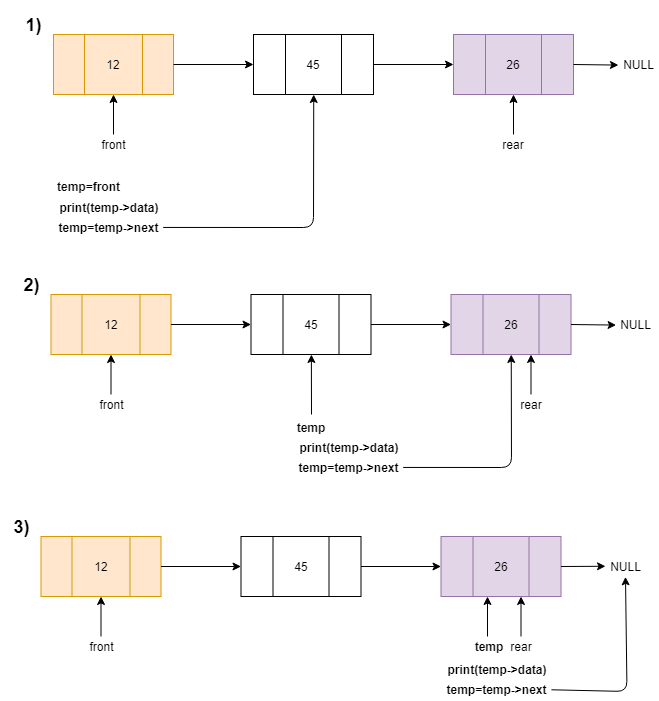``````Step 1 : Check whether queue is Empty (front == NULL).
Step 2 : If it is Empty then, display 'Queue is Empty!!!' and terminate the function.
Step 3 : If it is Not Empty then, define a Node pointer 'temp' and initialize with front.
Step 4 : Display 'temp → data -->' and move it to the next node. Repeat the same until 'temp' reaches to 'rear' (temp → next != NULL).
Step 5 : Finally! Display 'temp → data --> NULL'.

``````

# Complexity

• Time complexity of DeQueue and EnQueue operation is O(1),Because there is no loop in those operation.
• Time complexity of Display operation is O(n),Since we are traversing to print each n(number of elements) elements.
• Space complexity is O(n),Where n is number of elements.

# Implementation

Following is the implementation of Queue using Linked Lists in C:

``````#include<stdio.h>
#include<conio.h>

struct Node
{
int data;
struct Node *next;
};

struct Node *front = NULL,*rear = NULL;

void EnQueue(int);
void DeQueue();
void display();
void Front();
void QueueSize();
int main()
{
int choice, value;
printf("\n*** Queue Implementation using Linked List ***\n");
while(1)
{
printf("1. Insert in Queue\n");
printf("2. Delete From Queue\n");
printf("3. Display Queue\n");
printf("4. Front of the Queue\n");
printf("5. Size of Queue\n");
printf("6. Exit\n");

scanf("%d",&choice);

switch(choice)
{
case 1:
printf("Insert the value you want to enter: ");
scanf("%d", &value);

EnQueue(value);
break;
case 2:
DeQueue();
break;
case 3:
display();
break;
case 4:
Front();
break;
case 5:
QueueSize();
break;
case 6:
exit(0);
default:
};
}
return 0;
}

void EnQueue(int value)
{
struct Node *newNode;
newNode = (struct Node*)malloc(sizeof(struct Node));
newNode -> data = value;
newNode -> next = NULL;

if(front == NULL)
front = rear = newNode;
else
{
rear -> next = newNode;
rear = newNode;
}
printf("\n Data inserted in Queue!!!\n");
}

void DeQueue()
{
if(front == NULL)
printf("\n Queue is Empty!!!\n");
else
{
struct Node *temp = front;
front = front -> next;
printf("\n Deleted element is: %d\n", temp->data);
free(temp);
}
}

void display()
{
if(front == NULL)
printf("\n Queue is Empty!!!\n");
else
{
struct Node *temp = front;
while(temp->next != NULL)
{
printf("%d --> ",temp->data);
temp = temp -> next;
}
printf("%d \n",temp->data);
}
}

void Front()
{
if(front==NULL)
printf("\n Queue is Empty!!!\n");
else
printf("\n Data at front of the queue is %d \n",front->data);
}

void QueueSize()
{
if(front==NULL)
printf("\n Queue is Empty!!!\n");
else
{
int count=0;
struct Node *temp = front;
while(temp->next != NULL)
{
count++;
temp = temp -> next;
}
printf("\n Size of the queue is %d \n",count+1);
}
}
``````

### Implementation Using Linked list functions

The difference is that is this implementation we are using a linked list as a queue without the implementations of the common functions like enqueue and dequeue.

``````#include<stdio.h>
#include<conio.h>
struct Node{

int data;

};
struct Node* root=NULL;

void append();
void deletefirst();
void display();
void length();

int main()
{
int choice;
printf("\n*** Queue Implementation using Linked List Function ***\n");
while(1)
{
printf("1. Insert in Queue\n");
printf("2. Delete From Queue\n");
printf("3. Display Queue\n");
printf("4. Front of the Queue\n");
printf("5. Size of Queue\n");
printf("6. Exit\n");

scanf("%d",&choice);

switch(choice)
{
case 1: append();
break;
case 2: deletefirst();
break;
case 3: display();
break;
case 4: if(root==NULL)
printf("\n Queue is Empty!!!\n");
else
printf("\n Data at front of the queue is %d \n",root->data);
break;
case 5: length();
break;
case 6: exit(0);
default:
printf("\n invalid number \n");
}
}
return 0;
}

void append()
{
struct Node *temp;
temp=(struct Node*)malloc(sizeof(struct Node));
printf("Insert the value you want to enter: ");
scanf("%d",&temp->data);
if(root==NULL)
{
root=temp;
}
else
{
struct Node *p;
p=root;
{
}
}
printf("\n Data inserted in Queue!!!\n");
}

void display()
{
struct Node *temp;
temp=root;
if(root==NULL){
printf("\n Queue is Empty!!!\n");
return;
}
{
printf("%d --> ",temp->data);
}
printf("%d\n",temp->data);
}

void length()
{
struct Node *temp;
int count=0;
temp=root;
if(temp==NULL){
printf("\n Queue is Empty!!!\n");
return;
}
{
count++;
}
printf("\n Size of the queue is %d \n",count+1);
}

void deletefirst()
{
struct Node *x;
x=root;
if(root==NULL){
printf("\n Queue is Empty!!!\n");
return;
}
printf("\n Deleted element is: %d\n", root->data);
free(x);
}

``````

### Applications of Queue

1. In Operating system, scheduling processes and threads.
2. CPU scheduling, Disk Scheduling.
3. In Printer, Files will be in queue to be printed.
4. When data is transferred asynchronously (data not necessarily received at same rate as sent) between two processes. Examples include IO Buffers, pipes, file IO, etc.

## Question

#### To overcome the issue of the memory which of the following data structure is used to implement Queue?

Stack